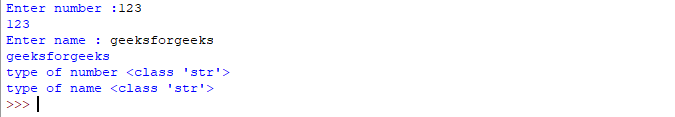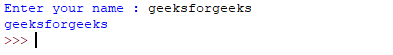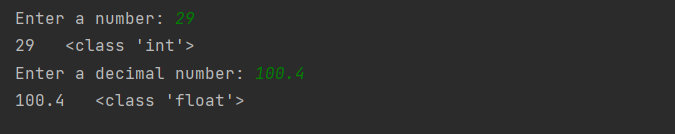Open in App
Not now

# Taking input in Python

• Difficulty Level : Easy
• Last Updated : 25 Dec, 2022

Developers often have a need to interact with users, either to get data or to provide some sort of result. Most programs today use a dialog box as a way of asking the user to provide some type of input. While Python provides us with two inbuilt functions to read the input from the keyboard.

• input ( prompt )
• raw_input ( prompt )

input (): This function first takes the input from the user and converts it into a string. The type of the returned object always will be <type ‘str’>. It does not evaluate the expression it just returns the complete statement as String. For example, Python provides a built-in function called input which takes the input from the user. When the input function is called it stops the program and waits for the user’s input. When the user presses enter, the program resumes and returns what the user typed.Syntax:

```inp = input('STATEMENT')

Example:
1.  >>> name = input('What is your name?\n')     # \n ---> newline  ---> It causes a line break
Ram
>>> print(name)
Ram

# ---> comment in python```

## Python3

 `# Python program showing ` `# a use of input()`   `val ``=` `input``(``"Enter your value: "``)` `print``(val)`

Output:Taking String as an input:

## Python3

 `name ``=` `input``(``'What is your name?\n'``)     ``# \n ---> newline  ---> It causes a line break` `print``(name)`

Output:

```What is your name?
Ram
Ram```

How the input function works in Python :

• When input() function executes program flow will be stopped until the user has given input.
• The text or message displayed on the output screen to ask a user to enter an input value is optional i.e. the prompt, which will be printed on the screen is optional.
• Whatever you enter as input, the input function converts it into a string. if you enter an integer value still input() function converts it into a string. You need to explicitly convert it into an integer in your code using typecastingCode:

## Python3

 `# Program to check input ` `# type in Python`   `num ``=` `input` `(``"Enter number :"``)` `print``(num)` `name1 ``=` `input``(``"Enter name : "``)` `print``(name1)`   `# Printing type of input value` `print` `(``"type of number"``, ``type``(num))` `print` `(``"type of name"``, ``type``(name1))`

Output:raw_input(): This function works in older version (like Python 2.x). This function takes exactly what is typed from the keyboard, converts it to string, and then returns it to the variable in which we want to store it.

Example:

## Python

 `# Python program showing` `# a use of raw_input()`   `g ``=` `raw_input``(``"Enter your name : "``)` `print` `g`

Output:Here, g is a variable that will get the string value, typed by the user during the execution of the program. Typing of data for the raw_input() function is terminated by enter key. We can use raw_input() to enter numeric data also. In that case, we use typecasting. For more details on typecasting refer this

Note: input() function takes all the input as a string only

There are various function that are used to take as desired input few of them are : –

• int(input())
• float(input())

## Python3

 `num ``=` `int``(``input``(``"Enter a number: "``))` `print``(num, ``" "``, ``type``(num))`   `          `  `floatNum ``=` `float``(``input``(``"Enter a decimal number: "``))` `print``(floatNum, ``" "``, ``type``(floatNum))`

Output:Output

Refer to the article Taking list as input from the user for more information.

My Personal Notes arrow_drop_up
Related Articles